Function Repository Resource:

# BulgarianStem

Find stems of Bulgarian words

Contributed by: Anton Antonov
 ResourceFunction["BulgarianStem"][word] gives a stem for the word word. ResourceFunction["BulgarianStem"][words] gives a stem for each of the words in the list words.

## Details

The algorithm of ResourceFunction["BulgarianStem"] is based on 130,000 stem rules.
The stems are essentially suffix replacements.
The largest suffixes are attempted first.
The character cases of the argument word are preserved in the stem.
Words without recognized suffixes by ResourceFunction["BulgarianStem"] are returned unchanged.
ResourceFunction["BulgarianStem"] takes arguments that allow control and monitoring of the stem rules that are applied.
There are three sets of rules that can be obtained with the argument "AllStemRulesWithCounts". The sets are kept in an association. The integers 1, 2, 3 are used as keys.
Stem rules are loaded with the (sub-value) function ResourceFunction["BulgarianStem"]["SetRules"[id,minCount]].
ResourceFunction["BulgarianStem"]["SetRules"[All,0]] loads all stem rules from all sets.
The (sub-value) function ResourceFunction["BulgarianStem"]["FetchRules"[id,minCount]] can be used to experiment with stem rules.
The function "SetRules" uses "FetchRules" in order to set the value of ResourceFunction["BulgarianStem"]["CurrentRules"].

## Examples

### Basic Examples (2)

Here is a stem or the word "качество":

 In:=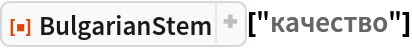Out=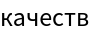Here are the stems for a list of words:

 In:=Out=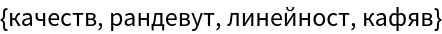### Scope (3)

The stem rules currently used by BulgarianStem can be retrieved with the argument "CurrentRules"; here is a sample of the current rules:

 In:=Out=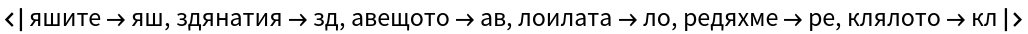Words without recognized suffixes by BulgarianStem are returned unchanged:

 In:=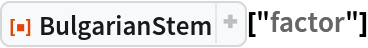Out=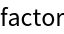The symbol BulgarianStem is overloaded—it takes arguments that allow the control and monitoring of the stem rules that are applied. There are three sets of rules.

The following command sets up the use of the third set with each rule having a frequency (count) of at least 2:

 In:=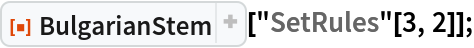Here is the number of rules (which were just set):

 In:=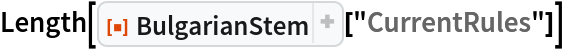Out=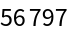Here is a sample of the rules:

 In:=Out=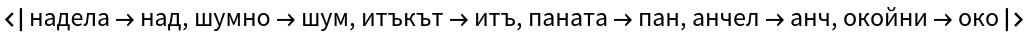Here are stems of the list of words above using the newly set rules:

 In:=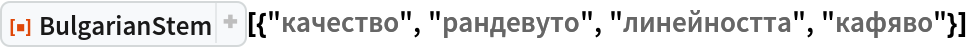Out=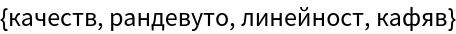Here we restore the default stem rules:

 In:=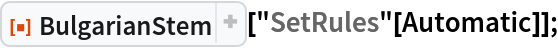### Applications (2)

Finding word stems is one of the fundamental procedures in information retrieval.

Take Bulgarian text from Wikipedia:

 In:=Here we get the words from the text:

 In:=Here we find the number of occurrences of each word and show the words with the largest counts:

 In:=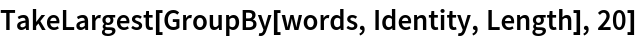Out=Here we stem the words, find the number of occurrences of each word stem and show the stems with the largest counts:

 In:=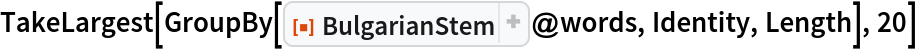Out=Consider the following random job titles in Bulgarian:

 In:=Out=Here is a list of tables that show the words of the job titles and their corresponding stems:

 In:=Out=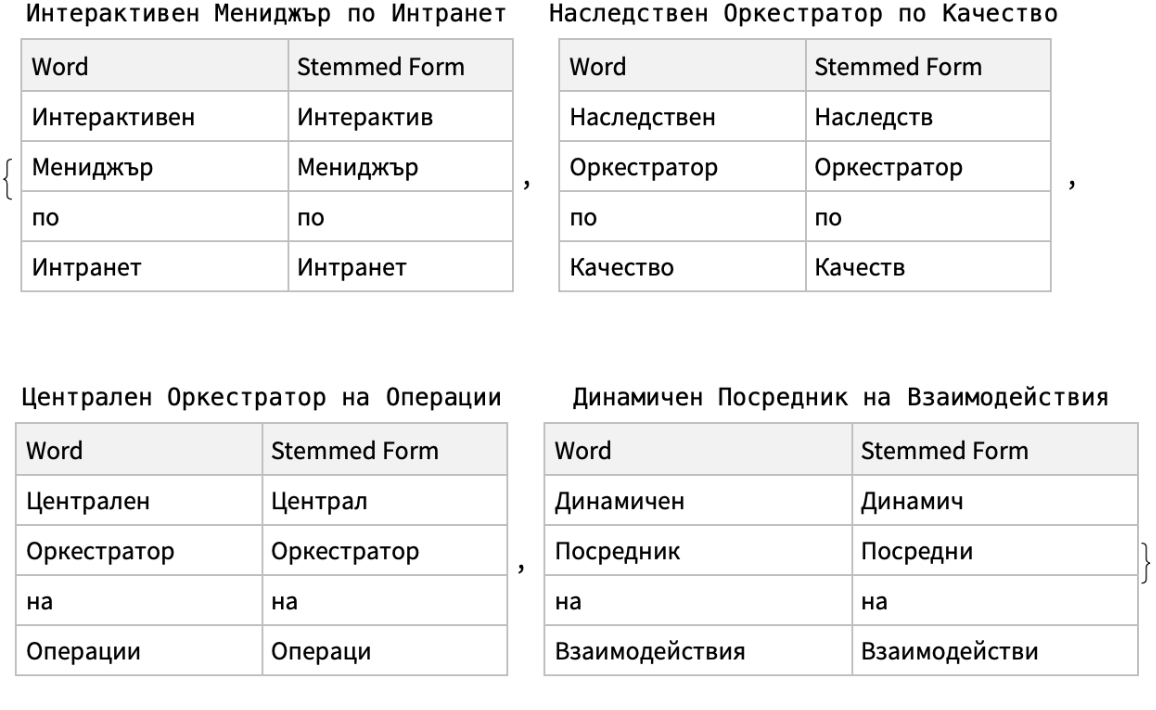### Properties and Relations (4)

Here is the number of rules in each of the three sets of rules:

 In:=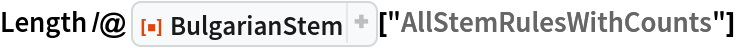Out=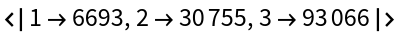Here are stem rule samples:

 In:=Out=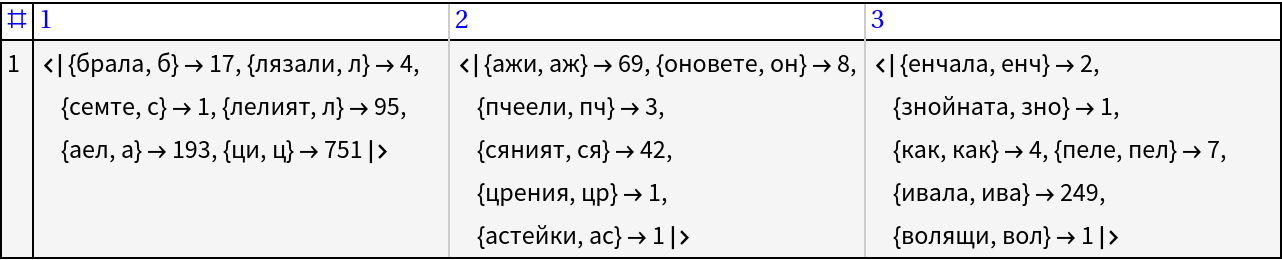The current set of stem rules can be obtained with the argument "CurrentRules":

 In:=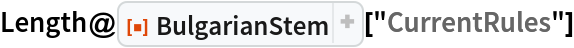Out=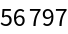Each stem rule has an associated count (or frequency); here is the minimum count of the current rules:

 In:=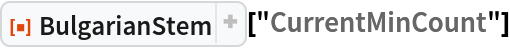Out=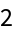Here is the number of current rules:

 In:=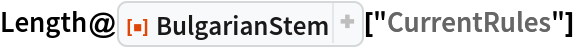Out=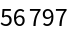Here is the string length of the replacement values of the current stem rules:

 In:=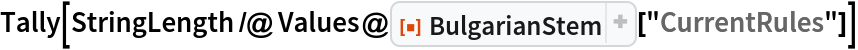Out=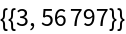Here is a histogram of the lengths of the suffixes that are replaced with the current stem rules:

 In:=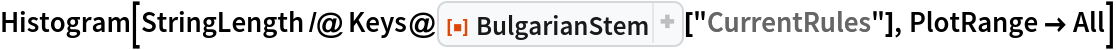Out=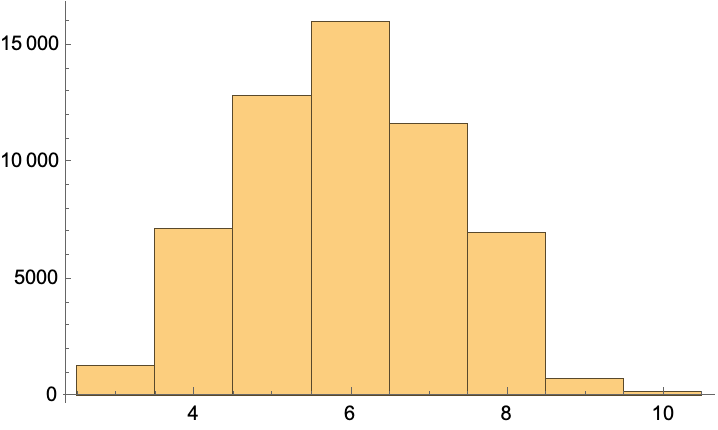The function WordStem gives stems of English words:

 In:=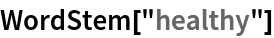Out=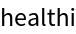Here is a corresponding call of BulgarianStem:

 In:=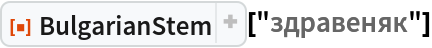Out=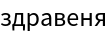Here is a list of tables that show the words of job titles in English and their corresponding stems (analogous to the list of tables above):

 In:=Out=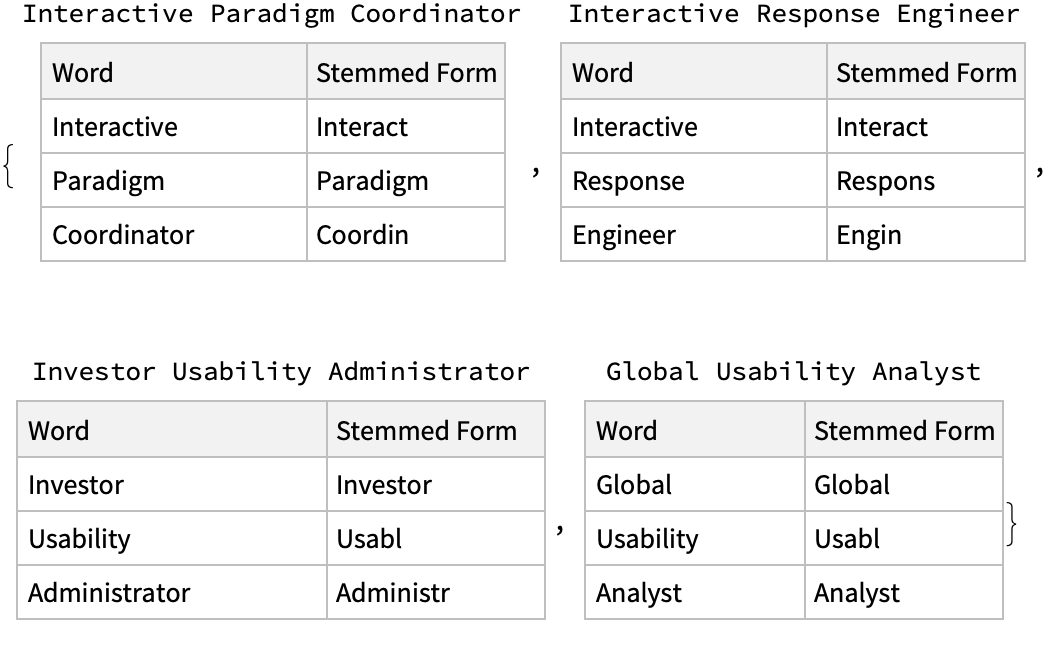The words of a text can be obtained with StringSplit or TextWords and then given to BulgarianStem:

 In:=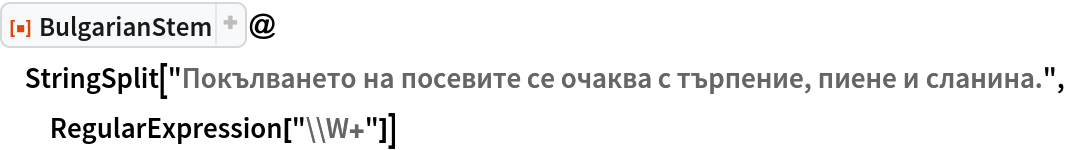Out=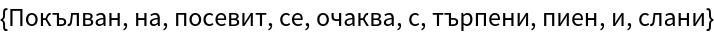### Neat Examples (3)

Here is some English text:

 In:=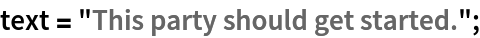Here are the stems of the words in the English text:

 In:=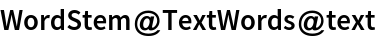Out=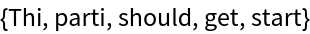Here we translate the English text into Bulgarian text, extract the words and stem them:

 In:=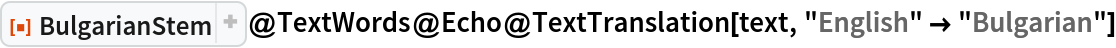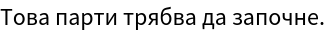Out=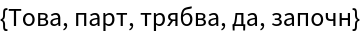Anton Antonov

## Version History

• 1.0.0 – 22 April 2022